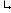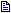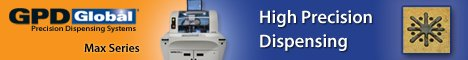Printed Circuit Board Assembly & PCB Design Forum

SMT electronics assembly manufacturing forum.

# Mathematical FormulaI rotated my PCB -90 degrees. Now what's the formula to ca... - Aug 24, 1999 by| I rotated my PCB -90 degrees. Now what's the formula to ... - Aug 24, 1999 by| | I rotated my PCB -90 degrees. Now what's the formula t... - Aug 26, 1999| | | I rotated my PCB -90 degrees. Now what's the formula... - Aug 26, 1999| | | I rotated my PCB -90 degrees. Now what's the formula... - Aug 27, 1999 by

Drake

Mathematical Formula | 24 August, 1999

I rotated my PCB -90 degrees. Now what's the formula to calculate the X-Y Coordinates of my component CAD Data?

Thanks,

Brian

Re: Mathematical Formula | 24 August, 1999

| I rotated my PCB -90 degrees. Now what's the formula to calculate the X-Y Coordinates of my component CAD Data? | | Thanks, | Not very difficult

(Subscripts n = new, o = old)

Assuming the same origin:

Yn = Xo Xn = -Yo

Brian

Mika Johnsson

Re: Mathematical Formula | 26 August, 1999

| | I rotated my PCB -90 degrees. Now what's the formula to calculate the X-Y Coordinates of my component CAD Data? | | | | Thanks, | | | Not very difficult | | (Subscripts n = new, o = old) | | Assuming the same origin: | | Yn = Xo | Xn = -Yo | | Brian | Hi !

I may have misunderstood the question (or assumptions), but in our software when we e.g. transform the coordinates from design coordinates to printing (machine) coordinates, and there exist an angle difference, also the board dimensions are needed in the calculation

so:

90 clockwise

Xn = Yo Yn = Xlength - Xo

90 degrees anticlockwise

Xn = Ylength - Yo Yn = Xo

Where Xlength and Ylength are board dimensions in the initial coordinate system.

Mika

Re: Mathematical Formula | 26 August, 1999

| | | I rotated my PCB -90 degrees. Now what's the formula to calculate the X-Y Coordinates of my component CAD Data? | | | | | | Thanks, | | | | | Not very difficult | | | | (Subscripts n = new, o = old) | | | | Assuming the same origin: | | | | Yn = Xo | | Xn = -Yo | | | | Brian | | | Hi ! | | I may have misunderstood the question (or assumptions), but in our software when we e.g. transform the coordinates from design coordinates to printing (machine) coordinates, and there exist an angle difference, also the board dimensions are needed in the calculation | | so: | | 90 clockwise | | Xn = Yo | Yn = Xlength - Xo | | 90 degrees anticlockwise | | Xn = Ylength - Yo | Yn = Xo | | Where Xlength and Ylength are board dimensions in the initial coordinate system. | | Mika | Just another thought, remembering the old times generating data for the machines I did not change the data all I had to do is tell the machine in which angle the PCB was going through. So if we made more than one unit simultaniously they often were not in the same angle and you could tell the specific angle for each unit. For one unit only we set the machines in multiple-unit-mode and the number of units to one. But I don�t know if it is possible with your equipment.

maybe it�ll help

Wolfgang

Brian

Re: Mathematical Formula | 27 August, 1999

| | | I rotated my PCB -90 degrees. Now what's the formula to calculate the X-Y Coordinates of my component CAD Data? | | | | | | Thanks, | | | | | Not very difficult | | | | (Subscripts n = new, o = old) | | | | Assuming the same origin: | | | | Yn = Xo | | Xn = -Yo | | | | Brian | | | Hi ! | | I may have misunderstood the question (or assumptions), but in our software when we e.g. transform the coordinates from design coordinates to printing (machine) coordinates, and there exist an angle difference, also the board dimensions are needed in the calculation | | so: | | 90 clockwise | | Xn = Yo | Yn = Xlength - Xo | | 90 degrees anticlockwise | | Xn = Ylength - Yo | Yn = Xo | | Where Xlength and Ylength are board dimensions in the initial coordinate system. | | Mika | This changes the origin, as well, which was not suggested.

Brian